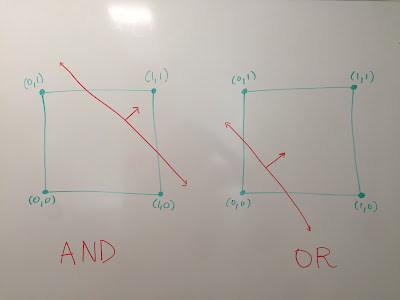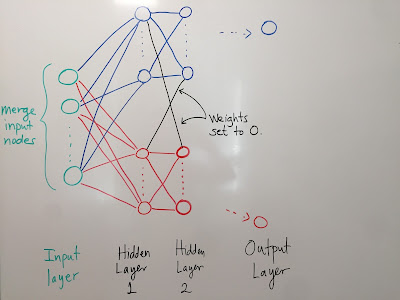# Neural networks extend boolean functions from the vertices to the interior of the unit n-cube

I'm fired up because I started a learning seminar on the mathematics of neural networks this semester at BC. Jeffery Breeding-Allison, a visiting assistant professor here who did the Insight Data Science Fellows program last summer, is my co-organizer.

We're focusing not as much on the practical computational aspects of neural networks as on the theoretical computer science/mathematical aspects. It's not that the practical aspects aren't important (they are, of course). It's just that at the moment I want to learn about the theoretical aspects.

And I do what I want. (Not really, but doesn't that sound fun?)

Before I start in on the math, let me just say: Man, it's nice to be talking regularly about this stuff with actual people in real life. Much of the perspective I take below (especially the connection to classical Morse theory) arose during chats with Nate B.

My goal for today is to convince you of the following cool fact.

Cool Fact 1 (CF1): Let m,nZ+. Any boolean function f:{0,1}n{0,1}m can be approximated arbitrarily well by a finite feedforward neural network with sigmoid activation functions.

More precisely, there exists a neural network with an n-node input layer and an m-node output layer whose associated smooth function F:Rn(0,1)m can be extended continuously to a function F:Rn[0,1]m that (approximately!) agrees with f on the vertices, {0,1}n, of the unit n-cube.

I should note first of all that this fact has been known for decades. Indeed, it is implied by the much harder universal approximation theorem for sigmoid feedforward neural networks (the google tells me this is attributed to Cybenko and Funahashi, who gave independent proofs both published in 1989). The universal approximation theorem says that any smooth function from (0,1)n(0,1)m can be approximated arbitrarily closely by a sufficiently large feedforward neural network with sigmoid activation functions.

What I like about the more modest statement in bold (CF1) above is that

• (CF1) is enough to tell you that, in principle, a sufficiently large trained neural network can at least do the sorts of calculations a computer program can. After all, at heart these calculations are nothing more than boolean functions.
• Finding a sensible way to extend a boolean function from the vertices of an n-cube to its interior is a beautiful thing to do, since you are essentially finding a probabilistic function that extends a deterministic one. That is, you're replacing binary ("Yes/No") inputs/outputs with inputs/outputs in the interval (0,1) ("maybe Yes, with probability p(0,1)").
• Understanding why (CF1) is true gets you a long way towards a rudimentary picture of how a neural network is "reasoning". And that's what we're after.
As a side note, I have to say that I also like (CF1) for personal reasons. I'm a low-dimensional topologist by training, so I'm fond of handlebodies.

We can think of (CF1) as a neural network incarnation of the following fact. If you have a background in Morse theory, it should be straightforward to supply a proof that does not appeal to (CF1):

Cool Fact 2 (CF2): Let m,nZ+, let Bn be the unit ball, and let P be a set of finitely many points on (Bn)=Sn1, the boundary of Bn.  Suppose S1,,Sm are m subsets of P. Then there exists a continuous function f=(f1,,fm):Bn[0,1]m that satisfies the property that fi sends Si to 1 and PSi to 0 for all i. Moreover, each fi can be assumed to be Morse.

Here's an example in the case n=2 and m=1:4 points on the boundary of a 2-dimensional disk, along with (the graph of) a Morse function to the unit interval that sends the blue points to 1 and the red points to 0. (The blue and red lines are there just to make the picture on the right easier to understand.) To believe (CF2) completely in the 2D case, imagine that the disk is made of pizza dough. Invite your friends to pick up all of the blue points and lift them to height 1 above a table. The red points will stay at height 0, and the pizza dough will look like the graph of a Morse function with the desired properties.

If we note that Bn is homeomorphic to the unit n-cube, [0,1]n, and we let P be the set of its vertices, {0,1}n([0,1]n), (CF1) is saying that we can construct the function in (CF2) using a neural network.

Now that we're feeling motivated, let's get to it.

There are only four things we need to understand for everything to fall into place.
1. Every boolean function {0,1}n{0,1} can be realized as a composition involving ANDs, NOTs, and ORs. (In fact, all you really need is NOT AND, often abbreviated NAND.)
2. ANDs, NOTs, and ORs can all be modeled by perceptrons.
3. Perceptrons can be approximated arbitrarily closely by sigmoid neurons.
4. An m-output neural network can be constructed by stacking m independent 1-output neural networks.
In a certain sense, the first three points are the takeaways from my previous post about perceptrons. I definitely didn't appreciate the implications at that time, though, so I'll start by re-explaining some things quickly, then put it all together at the end.

Thing 1: Every 1-output boolean function is a composition of ANDs, NOTs, and ORs

You can find this statement in the first chapter of any electrical engineering textbook, because it's the foundational fact underpinning circuit design. What does it mean?

Formally: Given any n-input, 1-output boolean function

f:{0,1}n{0,1}

(there are 22n possible such functions) we can realize f as a composition of AND, NOT, & OR functions on the inputs. Recall:
• The "AND" function takes two binary inputs and outputs a 1 iff both inputs are 1s. That is, if we have two binary inputs 1{0,1} and 2{0,1}, then (1 AND 2)=1 iff 1=2=1.
• The "OR" function takes two binary inputs and outputs a 0 iff both inputs are 0. That is, (1 OR 2)=0 iff 1=2=0.
• The "NOT" function takes a single binary input and flips it. So NOT()=1 iff =0.
For the purposes of the remaining discussion, let's use the standard abbreviations of:
• AND by
• OR by , and
• NOT by ¬.
OK, so why is it that we can realize any n-input boolean function as a composition of the simple binary functions , and ¬? Well, for a sort of stupid reason. One perfectly sensible way to describe a boolean function is just to provide a complete list of all of the inputs that map to 1. This is the idea behind the so-called canonical representation of a boolean function. The universal existence of the canonical representation of a boolean function is the proof of (S1) we'll describe here.

It's easiest (and clearest) to describe the canonical representation by example. So I'll do that.

Suppose we consider the boolean function {0,1}3{0,1} that maps (0,1,0) and (1,1,1)to 1 and every other binary 3-tuple to 0. This boolean function can be written as:

(¬(1)(2)¬(3))(123),

which of course looks like jibberish when you look at it like that, so I'll draw an electrical "circuit" picture of it instead:The canonical representation of the boolean functionthat maps (0,1,0) and (1,1,1) to 1, and all other binary 3-tuples to 0

If it still seems like jibberish, here's the point. For any fixed binary n-tuple (a1,,an), there is a unique expression in ANDs and AND NOTs that outputs 1 iff the input, (1,,n), is equal to (a1,,an). To create this expression, just concatenate the i's using AND when ai=1and using AND NOT when ai=0.

So, e.g., the function ¬(1)(2)¬(3) outputs 1 iff (1,2,3)=(0,1,0).

Now to form the canonical representation of a boolean function, just list all of the inputs that map to 1, form the expressions as above that uniquely identifies each of these inputs, then concatenate all of these expressions using ORs.

Thing 2: AND, NOT, and OR can be modeled by perceptrons

Thing 2 was the main point of my post about perceptrons. An n-input, 1-output perceptron uniquely specifies a function that assigns a 1 to one side of an affine hyperplane in Rn and a 0 to the other. Such functions are called threshold functions in the literature. Geometrically, the weights and bias associated to a perceptron are irrelevant, except insofar as they define the co-oriented affine hyperplane that separates 0 outputs from 1 outputs.

We can therefore understand Thing 2 by understanding the pair of statements:
• the 2-input boolean functions "AND" and "OR" can be realized by threshold functions, and
• we can realize the 1-input boolean function "NOT" by reversing the co-orientation of the affine hyperplane defining the threshold function.
The second statement of the pair is clear from the definition of a threshold function, and the first statement becomes clear once you stare at the following two pictures while recalling the definitions of "AND", "OR".The threshold functions that map the points on the right (arrow-side) of each of these red affinehyperplanes to 1 and the left (non-arrow-side) to 0 extend the 2-input boolean functions AND & OR

Thing 3: Perceptrons can be approximated arbitrarily closely by sigmoid neurons

Thing 3 was also explained briefly in my post about perceptrons (look at the pictures at the end, in the "And now back to that thought we were holding" section). The key observation is that the sigmoid functions

σ(t)=11+ect

are nice smooth approximations of the step function

S(t)={01if t<0if t0,

and these approximations get successively better as c.

Indeed, the sigmoid functions converge pointwise to the Heaviside step function (really a better choice for a step function anyway, because of its symmetry):

H(t)=0121if t<0if t=0if t>0

Once you understand this, all you need to appreciate is that an n-input sigmoid neuron is just a perceptron whose step function has been replaced by a sigmoid function.

More precisely, recall that a (co-oriented) affine hyperplane H in Rn is determined (non-uniquely) by a weight vector w⃗ Rn and a bias bR as follows:

Hw⃗ ,b={x⃗ Rn|w⃗ x⃗ b=0}.

The weight vector w⃗  is just a vector orthogonal to the hyperplane, pointing in the direction of the co-orientation. The bias bR determines the shift of this hyperplane from the origin (its absolute value is equal to the distance of H away from the origin in the case w⃗  has length 1).

With this understood, we now see that a threshold function is just the composition of the step function with an affine linear function. That is, the threshold function associated to Hw⃗ ,b is simply S(w⃗ x⃗ b). And this makes it clear why a corresponding sigmoid neuron σ(w⃗ x⃗ b) can be viewed as a smooth approximation to it. N.B.: this approximation can be made arbitrarily good by scaling w⃗  and b by the same large c>0.

Thing 4: We can construct an m-output neural network by "stacking" m independent 1-output NN's

This is a neural network analog of the statement that a function to a product can (should?) be defined component by component.

Informally, what I mean is the following. If you have m 1-output neural networks, all with the same n inputs, and you want to build a neural network whose output layer is precisely those moutputs, just stack the m 1-output neural networks vertically, then merge the m identical copies of the input layer. Here are some pictures, to illustrate what I mean:Two separate neural networks with the same inputs, each witha single output, drawn one above the otherThe two separate neural nets above have now been mergedinto a single one, with two outputs

The key to keeping these m parallel computations independent from each other is to force edges to have 0 weight whenever you don't want the output of the edge to depend on the input. Here are another couple of pictures, to illustrate what I mean by that:The blue nodes shouldn't interact with the red nodesSo we artificially set all weights on edges connecting them to zero

Putting it all together:

OK, that was long, so let's recap. We're trying to understand why a neural network is a robust enough architecture to extend any boolean function from the vertices to the interior of a unit n-cube. I.e., we're trying to sketch an argument of:

Cool Fact 1 (CF1): Let m,nZ+. Any boolean function f:{0,1}n{0,1}m can be approximated arbitrarily well by a finite feedforward neural network with sigmoid activation functions.

We proceed as follows. Look at the m components f1,,fm of f

Each of these is a 1-output boolean function, fi:{0,1}n{0,1}. So we can represent it as a composition of ANDs, NOTs, & ORs (e.g., by using the canonical representation, or- if we're lucky-in some other, more succinct, way). Now model each of these ANDs, NOTs, & ORs by threshold functions associated to perceptrons, then replace each perceptron by a sigmoid neuron that approximates it sufficiently closely. We now have m independent 1-output neural networks. Merge them into a single m-output neural network by merging the inputs and zeroing out weights on edges connecting nodes between different neural networks.

And there you have it! If I were less pooped out, I'd close with some further questions. But maybe I'll just leave it there for today.